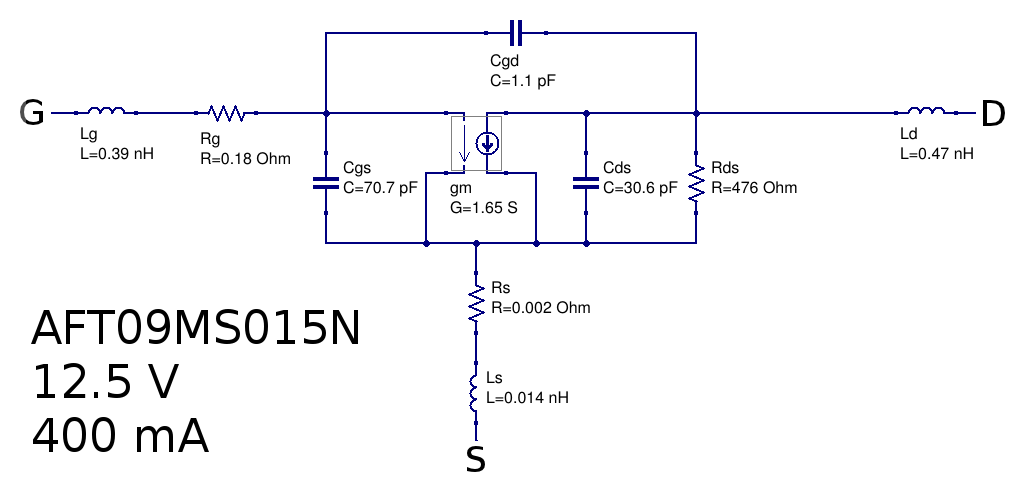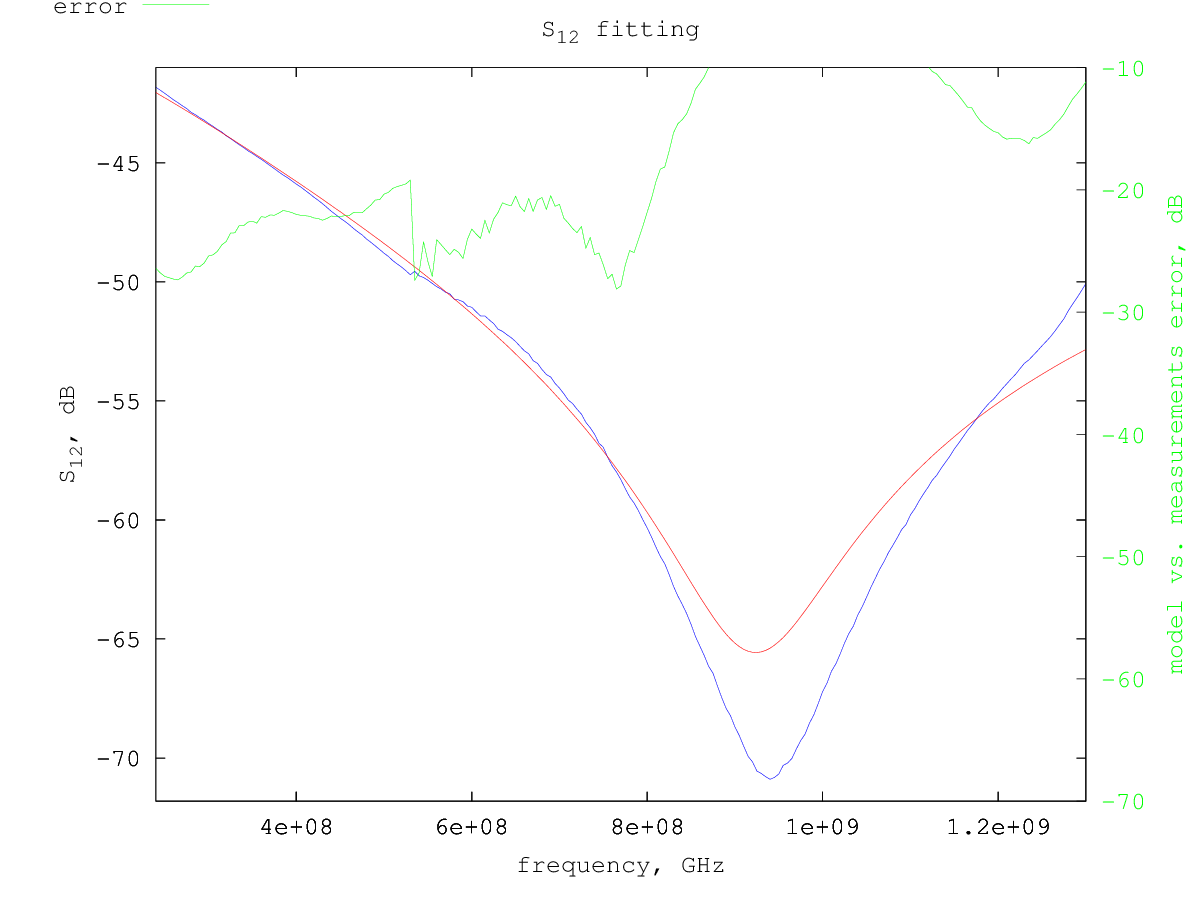# Freescale AFT09MS015N LDMOS model

Under construction...

The Freescale AFT09MS015N is a low power LDMOS.

# Model for Vdd=12.5 V and Idd= 400 mA

Typical S-parameters for this device can be found on the NXP/Freescale web site, for a supply voltage of 12.5 V and a drain current of 400 mA.

Using the extraction method previously described, considering only the S-parameters from 240 MHz to 1 GHz, the following small-signal model parameters are determined:

```Rg = 0.18 ohm
Rd = 0.0 ohm
Rs = 0.002 ohm
Lg = 0.39 nH
Ld = 0.47 nH
Ls = 0.014 nH
Cgs = 70.7 pF
Cds = 30.6 pF
Cgd = 1.1 pF
gm = 1.65 S
gds = 2.1 mS
```
So the equivalent small-signal circuit for this LDMOS at 400 mA drain current is the following:Smith chart with measured vs. model S-parameters:S-parameters fitting details - fitted region is in white background: